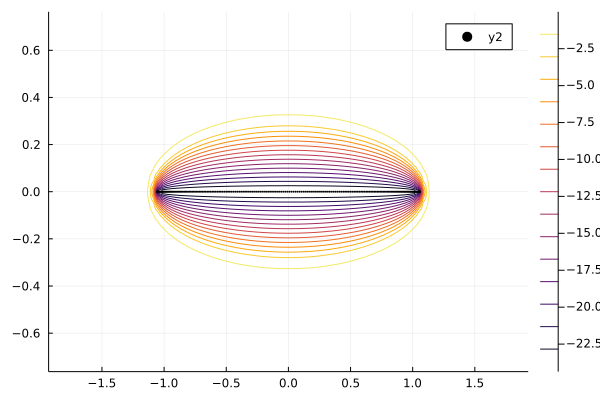# Tridiagonal Toeplitz matrix complex eigenvalues instead of real

The spectrum of tridiagonal Toeplitz matrices is known analytically (Tridiagonal-Toeplitz-Matrix – Wikipedia) and is (for non-zero off-diagonals) simple and real. Julia’s eigen function returns for small non-symmetric Toeplitz matrices the correct real spectrum, but for larger sizes fails to do so. E.g.

``````n = 200
p = 0.7
q = 0.4
A = Tridiagonal([q for _ in 1:(n-1)], zeros(n), [p for _ in 1:(n-1)])
A = Array(A)
maximum(imag.(eigvals(A)))
``````

Shows non-zero imaginary parts significantly larger than machine precision. The same happens in numpy. Changing to BigFloat helped for sizes of n around 200. I did not test for larger n, but would guess that at sufficiently large n it will fail as well.

Is there a way to detect the failure of `eigen`?

EDIT: In python an example was given here: python - How to get real eigenvalues and eigenvectors for a tridiagonal Toeplitz matrix? - Stack Overflow
and here
python - Eigenvectors are complex but only for large matrices - Stack Overflow

2 Likes

The eigenvalues of these matrices are super ill conditioned. I spent some time on these matrices with @sverek a while ago. Sven-Erik knows a lot more about these than I do.

The condition number of the eigenvalue is roughly reciprocal of the inner product between the right and the left (normalized) eigenvectors for that value. (I think I have the condition number from Nick HIgham’s book). So you have

``````julia> n = 20;

julia> A = Tridiagonal([q for _ in 1:(n-1)], zeros(n), [p for _ in 1:(n-1)]);

julia> F = eigen(Array(A));

julia> vr = (A - F.values[end]*I)\randn(n) |> t -> t/norm(t);

julia> vl = (A' - F.values[end]*I)\randn(n) |> t -> t/norm(t);

julia> abs(inv(vl'vr))
6.737730466564851

julia> n = 200;

julia> A = Tridiagonal([q for _ in 1:(n-1)], zeros(n), [p for _ in 1:(n-1)]);

julia> F = eigen(Array(A));

julia> vr = (A - F.values[end]*I)\randn(n) |> t -> t/norm(t);

julia> vl = (A' - F.values[end]*I)\randn(n) |> t -> t/norm(t);

julia> abs(inv(vl'vr))
7.360334831197046e19
``````

so `n` doen’t have to get large before small perturbations will be heavily amplified in the eigenvalues.

3 Likes

I can send you some current references regarding this (and some ways how to deal with it). By the way, the spectrum does not have to be real (e.g., just set q=-0.4).

1 Like

By the way, when I run your code

``````using LinearAlgebra
n = 200
p = 0.7
q = 0.4
A = Tridiagonal([q for _ in 1:(n-1)], zeros(n), [p for _ in 1:(n-1)])
A = Array(A)
``````

to build the matrix `A` of the size 200×200 in Julia 1.6.3 and then I compute the eigenvalues, I get just real eigenvalues for this size

``````julia> λ = eigvals(A)
200-element Vector{Float64}:
-1.058223000149799
-1.0576000083075798
-1.0572843720725433
-1.056332059729569
-1.0544327076739584
-1.0543669353419873
-1.0515818708879903
-1.0503080759555965
-1.0477502207464444
-1.045428090444631
⋮
1.0477851444774355
1.050211862207378
1.051655860894167
1.0543900728172997
1.0543919786468086
1.0563388912151381
1.0572818987403343
1.0576011289599778
1.058222590801755
``````

I only get complex eigvenvalues for `n=207` (and larger).

1 Like

Fyi, that is not the case here on Win10 for Julia 1.6.3 or v"1.7.0-rc1" …

NB:
the maximum imaginary part is ~ 0.05% of the corresponding real part

1 Like

How come? Julia is the same, the rules for floating point arithmetics are the same. Is it that it is linked to different BLAS or LAPACK or what?

2 Likes

As @andreasnoack mentions, the matrix is ill-conditioned. For such matrices, computing pseudospectrum instead of spectrum (eigenvalues) gives some/more insight. Julia has a package for computing Pseudospectra too.

For your matrix with `n=1000` it looks like thisThe code to produce it this:

``````using LinearAlgebra
using Plots
using Pseudospectra

n = 1000
p = 0.7
q = 0.4
A = Tridiagonal([q for _ in 1:(n-1)], zeros(n), [p for _ in 1:(n-1)])
A = Array(A)
λ = eigvals(A)

spectralportrait(A)
``````

For `n=200`, the pseudospectrum is this2 Likes

Thank you for all your great answers. I was not aware that finding the eigenvalues of tridiagonal Toeplitz matrices is such an ill-conditioned problem. I will mark @andreasnoack post as answer, but I am very grateful for all your comments, especially @zdenek_hurak pointing to the pseudo spectrum.

@zdenek_hurak @rafael.guerra I did my tests in Windows 10. On WSL 2, Ubuntu 20.04 I get non-zero imaginary parts for `n=200` as well. My specs are for
Windows

``````Julia Version 1.6.2
Commit 1b93d53fc4 (2021-07-14 15:36 UTC)
Platform Info:
OS: Windows (x86_64-w64-mingw32)
CPU: Intel(R) Core(TM) i7-8665U CPU @ 1.90GHz
WORD_SIZE: 64
LIBM: libopenlibm
LLVM: libLLVM-11.0.1 (ORCJIT, skylake)
Environment:
``````

and WSL 2 Ubuntu:

``````julia> versioninfo()
Julia Version 1.6.3
Commit ae8452a9e0 (2021-09-23 17:34 UTC)
Platform Info:
OS: Linux (x86_64-pc-linux-gnu)
CPU: Intel(R) Core(TM) i7-8665U CPU @ 1.90GHz
WORD_SIZE: 64
LIBM: libopenlibm
LLVM: libLLVM-11.0.1 (ORCJIT, skylake)
``````

Could it be something processor specific?